# Mean State

Period Mean (original grids) [Watt m-2]
Model Period Mean (intersection) [Watt m-2]
Model Period Mean (complement) [Watt m-2]
Benchmark Period Mean (intersection) [Watt m-2]
Benchmark Period Mean (complement) [Watt m-2]
Bias [Watt m-2]
RMSE [Watt m-2]
Phase Shift [months]
Bias Score 
RMSE Score 
Seasonal Cycle Score 
Spatial Distribution Score 
Interannual Variability Score 
Overall Score 
Benchmark [-] 217.
CLM5PHSOFF [-] 217. 217. 0.00 217. 232. 0.504 14.1 0.318 0.86 0.54 0.96 1.0 0.76 0.78
CLM5PHSON [-] 217. 216. 0.00 217. 232. 0.504 14.1 0.318 0.86 0.54 0.96 1.0 0.76 0.78
Period Mean (original grids) [Watt m-2]
Model Period Mean (intersection) [Watt m-2]
Model Period Mean (complement) [Watt m-2]
Benchmark Period Mean (intersection) [Watt m-2]
Benchmark Period Mean (complement) [Watt m-2]
Bias [Watt m-2]
RMSE [Watt m-2]
Phase Shift [months]
Bias Score 
RMSE Score 
Seasonal Cycle Score 
Spatial Distribution Score 
Interannual Variability Score 
Overall Score 
Benchmark [-] 241.
CLM5PHSOFF [-] 240. 241. 0.00 241. 238. -0.171 13.3 0.143 0.90 0.61 0.99 1.0 0.82 0.82
CLM5PHSON [-] 240. 241. 0.00 241. 238. -0.171 13.3 0.143 0.90 0.61 0.99 1.0 0.82 0.82
Period Mean (original grids) [Watt m-2]
Model Period Mean (intersection) [Watt m-2]
Model Period Mean (complement) [Watt m-2]
Benchmark Period Mean (intersection) [Watt m-2]
Benchmark Period Mean (complement) [Watt m-2]
Bias [Watt m-2]
RMSE [Watt m-2]
Phase Shift [months]
Bias Score 
RMSE Score 
Seasonal Cycle Score 
Spatial Distribution Score 
Interannual Variability Score 
Overall Score 
Benchmark [-] 233.
CLM5PHSOFF [-] 233. 233. 0.00 233. 238. 0.143 15.9 0.237 0.89 0.60 0.97 0.99 0.70 0.79
CLM5PHSON [-] 233. 233. 0.00 233. 238. 0.143 15.9 0.237 0.89 0.60 0.97 0.99 0.70 0.79
Period Mean (original grids) [Watt m-2]
Model Period Mean (intersection) [Watt m-2]
Model Period Mean (complement) [Watt m-2]
Benchmark Period Mean (intersection) [Watt m-2]
Benchmark Period Mean (complement) [Watt m-2]
Bias [Watt m-2]
RMSE [Watt m-2]
Phase Shift [months]
Bias Score 
RMSE Score 
Seasonal Cycle Score 
Spatial Distribution Score 
Interannual Variability Score 
Overall Score 
Benchmark [-] 111.
CLM5PHSOFF [-] 94.0 107. 0.00 111. 117. -3.90 12.6 0.0241 0.95 0.85 1.0 0.90 0.69 0.87
CLM5PHSON [-] 107. 107. 0.00 111. 117. -3.90 12.6 0.0241 0.95 0.85 1.0 0.90 0.69 0.87
Period Mean (original grids) [Watt m-2]
Model Period Mean (intersection) [Watt m-2]
Model Period Mean (complement) [Watt m-2]
Benchmark Period Mean (intersection) [Watt m-2]
Benchmark Period Mean (complement) [Watt m-2]
Bias [Watt m-2]
RMSE [Watt m-2]
Phase Shift [months]
Bias Score 
RMSE Score 
Seasonal Cycle Score 
Spatial Distribution Score 
Interannual Variability Score 
Overall Score 
Benchmark [-] 213.
CLM5PHSOFF [-] 210. 209. 0.00 209. 225. 0.417 17.6 0.524 0.79 0.46 0.92 0.99 0.77 0.73
CLM5PHSON [-] 205. 205. 0.00 209. 225. 0.417 17.6 0.524 0.79 0.46 0.92 0.99 0.77 0.73
Period Mean (original grids) [Watt m-2]
Model Period Mean (intersection) [Watt m-2]
Model Period Mean (complement) [Watt m-2]
Benchmark Period Mean (intersection) [Watt m-2]
Benchmark Period Mean (complement) [Watt m-2]
Bias [Watt m-2]
RMSE [Watt m-2]
Phase Shift [months]
Bias Score 
RMSE Score 
Seasonal Cycle Score 
Spatial Distribution Score 
Interannual Variability Score 
Overall Score 
Benchmark [-] 126.
CLM5PHSOFF [-] 126. 126. 0.00 126. 145. 0.0721 11.8 0.0425 0.98 0.86 1.0 1.0 0.64 0.89
CLM5PHSON [-] 126. 126. 0.00 126. 145. 0.0721 11.8 0.0425 0.98 0.86 1.0 1.0 0.64 0.89
Period Mean (original grids) [Watt m-2]
Model Period Mean (intersection) [Watt m-2]
Model Period Mean (complement) [Watt m-2]
Benchmark Period Mean (intersection) [Watt m-2]
Benchmark Period Mean (complement) [Watt m-2]
Bias [Watt m-2]
RMSE [Watt m-2]
Phase Shift [months]
Bias Score 
RMSE Score 
Seasonal Cycle Score 
Spatial Distribution Score 
Interannual Variability Score 
Overall Score 
Benchmark [-] 170.
CLM5PHSOFF [-] 171. 170. 0.00 170. 169. 0.441 14.1 0.129 0.95 0.79 0.99 1.0 0.62 0.85
CLM5PHSON [-] 171. 171. 0.00 170. 169. 0.441 14.1 0.129 0.95 0.79 0.99 1.0 0.62 0.85
Period Mean (original grids) [Watt m-2]
Model Period Mean (intersection) [Watt m-2]
Model Period Mean (complement) [Watt m-2]
Benchmark Period Mean (intersection) [Watt m-2]
Benchmark Period Mean (complement) [Watt m-2]
Bias [Watt m-2]
RMSE [Watt m-2]
Phase Shift [months]
Bias Score 
RMSE Score 
Seasonal Cycle Score 
Spatial Distribution Score 
Interannual Variability Score 
Overall Score 
Benchmark [-] 210.
CLM5PHSOFF [-] 209. 209. 0.00 209. 224. 0.482 16.5 0.148 0.89 0.66 0.99 0.99 0.66 0.81
CLM5PHSON [-] 208. 208. 0.00 209. 224. 0.482 16.5 0.148 0.89 0.66 0.99 0.99 0.66 0.81
Period Mean (original grids) [Watt m-2]
Model Period Mean (intersection) [Watt m-2]
Model Period Mean (complement) [Watt m-2]
Benchmark Period Mean (intersection) [Watt m-2]
Benchmark Period Mean (complement) [Watt m-2]
Bias [Watt m-2]
RMSE [Watt m-2]
Phase Shift [months]
Bias Score 
RMSE Score 
Seasonal Cycle Score 
Spatial Distribution Score 
Interannual Variability Score 
Overall Score 
Benchmark [-] 105.
CLM5PHSOFF [-] 95.6 95.8 0.00 105. 108. -8.76 12.5 0.0632 0.90 0.83 1.0 0.80 0.64 0.83
CLM5PHSON [-] 96.3 96.5 0.00 105. 108. -8.76 12.5 0.0632 0.90 0.83 1.0 0.80 0.64 0.83
Period Mean (original grids) [Watt m-2]
Model Period Mean (intersection) [Watt m-2]
Model Period Mean (complement) [Watt m-2]
Benchmark Period Mean (intersection) [Watt m-2]
Benchmark Period Mean (complement) [Watt m-2]
Bias [Watt m-2]
RMSE [Watt m-2]
Phase Shift [months]
Bias Score 
RMSE Score 
Seasonal Cycle Score 
Spatial Distribution Score 
Interannual Variability Score 
Overall Score 
Benchmark [-] 228.
CLM5PHSOFF [-] 229. 229. 0.00 229. 216. 0.0913 15.0 0.0879 0.95 0.76 0.99 1.0 0.68 0.86
CLM5PHSON [-] 232. 232. 0.00 229. 216. 0.0913 15.0 0.0879 0.95 0.76 0.99 1.0 0.68 0.86
Period Mean (original grids) [Watt m-2]
Model Period Mean (intersection) [Watt m-2]
Model Period Mean (complement) [Watt m-2]
Benchmark Period Mean (intersection) [Watt m-2]
Benchmark Period Mean (complement) [Watt m-2]
Bias [Watt m-2]
RMSE [Watt m-2]
Phase Shift [months]
Bias Score 
RMSE Score 
Seasonal Cycle Score 
Spatial Distribution Score 
Interannual Variability Score 
Overall Score 
Benchmark [-] 140.
CLM5PHSOFF [-] 138. 138. 0.00 138. 161. 0.0454 13.3 0.0808 0.95 0.85 0.99 1.0 0.64 0.88
CLM5PHSON [-] 135. 135. 0.00 138. 161. 0.0454 13.3 0.0808 0.95 0.85 0.99 1.0 0.64 0.88
Period Mean (original grids) [Watt m-2]
Model Period Mean (intersection) [Watt m-2]
Model Period Mean (complement) [Watt m-2]
Benchmark Period Mean (intersection) [Watt m-2]
Benchmark Period Mean (complement) [Watt m-2]
Bias [Watt m-2]
RMSE [Watt m-2]
Phase Shift [months]
Bias Score 
RMSE Score 
Seasonal Cycle Score 
Spatial Distribution Score 
Interannual Variability Score 
Overall Score 
Benchmark [-] 191.
CLM5PHSOFF [-] 190. 181. 0.00 186. 193. -4.43 14.4 0.132 0.91 0.73 0.99 0.99 0.66 0.83
CLM5PHSON [-] 182. 182. 0.00 186. 193. -4.43 14.4 0.132 0.91 0.73 0.99 0.99 0.66 0.83
Period Mean (original grids) [Watt m-2]
Model Period Mean (intersection) [Watt m-2]
Model Period Mean (complement) [Watt m-2]
Benchmark Period Mean (intersection) [Watt m-2]
Benchmark Period Mean (complement) [Watt m-2]
Bias [Watt m-2]
RMSE [Watt m-2]
Phase Shift [months]
Bias Score 
RMSE Score 
Seasonal Cycle Score 
Spatial Distribution Score 
Interannual Variability Score 
Overall Score 
Benchmark [-] 240.
CLM5PHSOFF [-] 240. 240. 0.00 240. 241. -0.0717 13.2 0.119 0.94 0.79 0.99 1.0 0.62 0.86
CLM5PHSON [-] 240. 240. 0.00 240. 241. -0.0717 13.2 0.119 0.94 0.79 0.99 1.0 0.62 0.86
Period Mean (original grids) [Watt m-2]
Model Period Mean (intersection) [Watt m-2]
Model Period Mean (complement) [Watt m-2]
Benchmark Period Mean (intersection) [Watt m-2]
Benchmark Period Mean (complement) [Watt m-2]
Bias [Watt m-2]
RMSE [Watt m-2]
Phase Shift [months]
Bias Score 
RMSE Score 
Seasonal Cycle Score 
Spatial Distribution Score 
Interannual Variability Score 
Overall Score 
Benchmark [-] 113.
CLM5PHSOFF [-] 108. 107. 0.00 113. 116. -5.10 12.3 0.0429 0.94 0.85 1.0 0.85 0.72 0.86
CLM5PHSON [-] 108. 108. 0.00 113. 116. -5.10 12.3 0.0429 0.94 0.85 1.0 0.85 0.72 0.86
Period Mean (original grids) [Watt m-2]
Model Period Mean (intersection) [Watt m-2]
Model Period Mean (complement) [Watt m-2]
Benchmark Period Mean (intersection) [Watt m-2]
Benchmark Period Mean (complement) [Watt m-2]
Bias [Watt m-2]
RMSE [Watt m-2]
Phase Shift [months]
Bias Score 
RMSE Score 
Seasonal Cycle Score 
Spatial Distribution Score 
Interannual Variability Score 
Overall Score 
Benchmark [-] 229.
CLM5PHSOFF [-] 227. 227. 0.00 227. 241. 0.355 16.5 0.210 0.85 0.68 0.98 0.99 0.69 0.81
CLM5PHSON [-] 226. 226. 0.00 227. 241. 0.355 16.5 0.210 0.85 0.68 0.98 0.99 0.69 0.81
Period Mean (original grids) [Watt m-2]
Model Period Mean (intersection) [Watt m-2]
Model Period Mean (complement) [Watt m-2]
Benchmark Period Mean (intersection) [Watt m-2]
Benchmark Period Mean (complement) [Watt m-2]
Bias [Watt m-2]
RMSE [Watt m-2]
Phase Shift [months]
Bias Score 
RMSE Score 
Seasonal Cycle Score 
Spatial Distribution Score 
Interannual Variability Score 
Overall Score 
Benchmark [-] 173.
CLM5PHSOFF [-] 173. 173. 0.00 172. 207. 0.686 13.8 0.0926 0.96 0.80 0.99 1.0 0.63 0.87
CLM5PHSON [-] 171. 171. 0.00 172. 207. 0.686 13.8 0.0926 0.96 0.80 0.99 1.0 0.63 0.87
Period Mean (original grids) [Watt m-2]
Model Period Mean (intersection) [Watt m-2]
Model Period Mean (complement) [Watt m-2]
Benchmark Period Mean (intersection) [Watt m-2]
Benchmark Period Mean (complement) [Watt m-2]
Bias [Watt m-2]
RMSE [Watt m-2]
Phase Shift [months]
Bias Score 
RMSE Score 
Seasonal Cycle Score 
Spatial Distribution Score 
Interannual Variability Score 
Overall Score 
Benchmark [-] 135.
CLM5PHSOFF [-] 135. 135. 0.00 135. 133. 0.0758 11.1 0.0540 0.98 0.86 1.0 1.0 0.65 0.89
CLM5PHSON [-] 135. 135. 0.00 135. 133. 0.0758 11.1 0.0540 0.98 0.86 1.0 1.0 0.65 0.89
Period Mean (original grids) [Watt m-2]
Model Period Mean (intersection) [Watt m-2]
Model Period Mean (complement) [Watt m-2]
Benchmark Period Mean (intersection) [Watt m-2]
Benchmark Period Mean (complement) [Watt m-2]
Bias [Watt m-2]
RMSE [Watt m-2]
Phase Shift [months]
Bias Score 
RMSE Score 
Seasonal Cycle Score 
Spatial Distribution Score 
Interannual Variability Score 
Overall Score 
Benchmark [-] 193.
CLM5PHSOFF [-] 193. 193. 0.00 193. 181. -0.0247 13.4 0.0748 0.97 0.84 1.0 1.0 0.65 0.88
CLM5PHSON [-] 193. 193. 0.00 193. 181. -0.0247 13.4 0.0748 0.97 0.84 1.0 1.0 0.65 0.88
Period Mean (original grids) [Watt m-2]
Model Period Mean (intersection) [Watt m-2]
Model Period Mean (complement) [Watt m-2]
Benchmark Period Mean (intersection) [Watt m-2]
Benchmark Period Mean (complement) [Watt m-2]
Bias [Watt m-2]
RMSE [Watt m-2]
Phase Shift [months]
Bias Score 
RMSE Score 
Seasonal Cycle Score 
Spatial Distribution Score 
Interannual Variability Score 
Overall Score 
Benchmark [-] 202.
CLM5PHSOFF [-] 202. 202. 0.00 201. 241. 0.303 15.0 0.334 0.86 0.50 0.96 0.99 0.75 0.76
CLM5PHSON [-] 201. 201. 0.00 201. 241. 0.303 15.0 0.334 0.86 0.50 0.96 0.99 0.75 0.76
Period Mean (original grids) [Watt m-2]
Model Period Mean (intersection) [Watt m-2]
Model Period Mean (complement) [Watt m-2]
Benchmark Period Mean (intersection) [Watt m-2]
Benchmark Period Mean (complement) [Watt m-2]
Bias [Watt m-2]
RMSE [Watt m-2]
Phase Shift [months]
Bias Score 
RMSE Score 
Seasonal Cycle Score 
Spatial Distribution Score 
Interannual Variability Score 
Overall Score 
Benchmark [-] 211.
CLM5PHSOFF [-] 212. 212. 0.00 211. 216. 0.782 17.8 0.173 0.92 0.62 0.98 1.0 0.67 0.80
CLM5PHSON [-] 212. 212. 0.00 211. 216. 0.782 17.8 0.173 0.92 0.62 0.98 1.0 0.67 0.80
Period Mean (original grids) [Watt m-2]
Model Period Mean (intersection) [Watt m-2]
Model Period Mean (complement) [Watt m-2]
Benchmark Period Mean (intersection) [Watt m-2]
Benchmark Period Mean (complement) [Watt m-2]
Bias [Watt m-2]
RMSE [Watt m-2]
Phase Shift [months]
Bias Score 
RMSE Score 
Seasonal Cycle Score 
Spatial Distribution Score 
Interannual Variability Score 
Overall Score 
Benchmark [-] 204.
CLM5PHSOFF [-] 206. 205. 0.00 205. 190. -0.797 19.6 0.252 0.88 0.73 0.97 1.0 0.64 0.82
CLM5PHSON [-] 210. 208. 0.00 205. 190. -0.797 19.6 0.252 0.88 0.73 0.97 1.0 0.64 0.82

# Temporally integrated period mean

BENCHMARK MEAN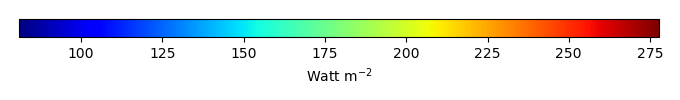MODEL MEANBIAS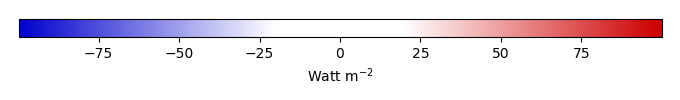BIAS SCORERMSE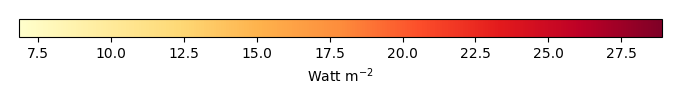RMSE SCOREBENCHMARK INTERANNUAL VARIABILITY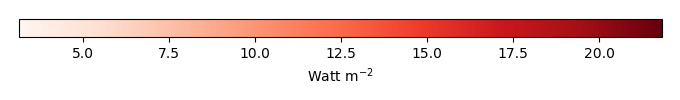MODEL INTERANNUAL VARIABILITYINTERANNUAL VARIABILITY SCOREBENCHMARK MAX MONTHMODEL MAX MONTHDIFFERENCE IN MAX MONTH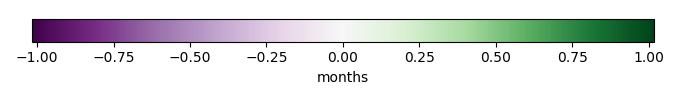SEASONAL CYCLE SCORESPATIAL TAYLOR DIAGRAMMODEL COLORS# Spatially integrated regional mean

MODEL COLORSREGIONAL MEANANNUAL CYCLEMONTHLY ANOMALYANNUAL CYCLE# All Models

BenchmarkCLM5PHSOFFCLM5PHSON# Data Information

creation_date: Mon Jun 30 23:16:59 PDT 2014

source_file: This product is generated from monthly 1 degree GEWEX SRB Radiation observations